Univerza na Primorskem Fakulteta za matematiko, naravoslovje in informacijske tehnologije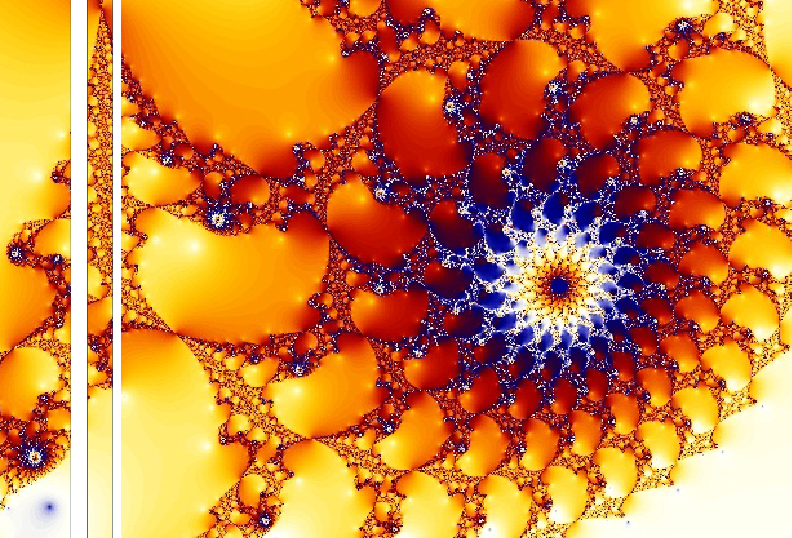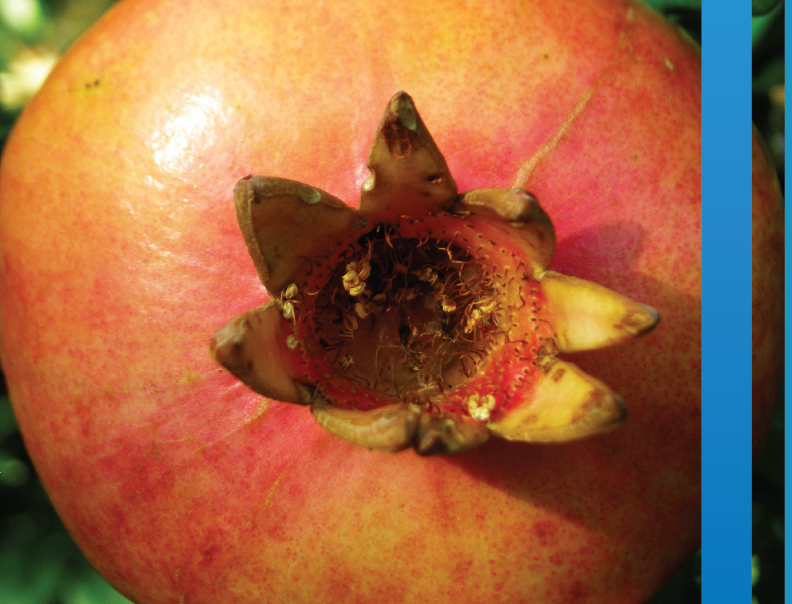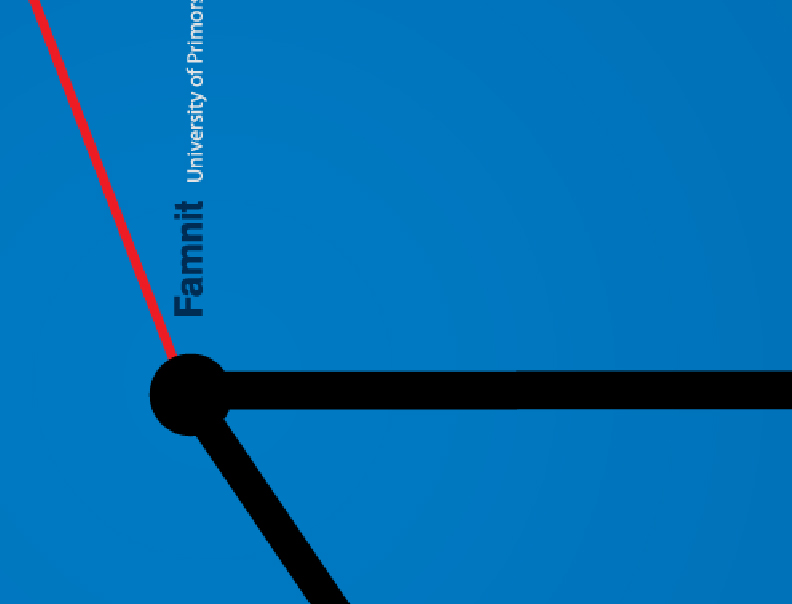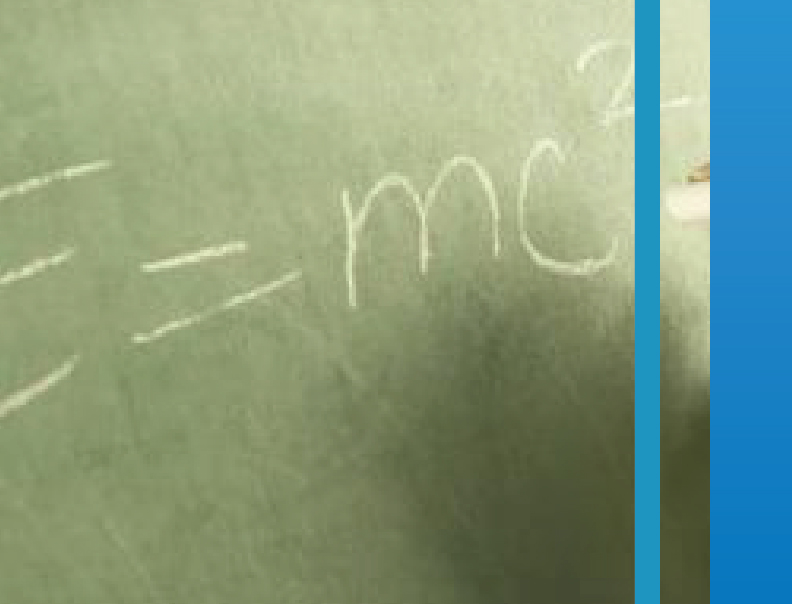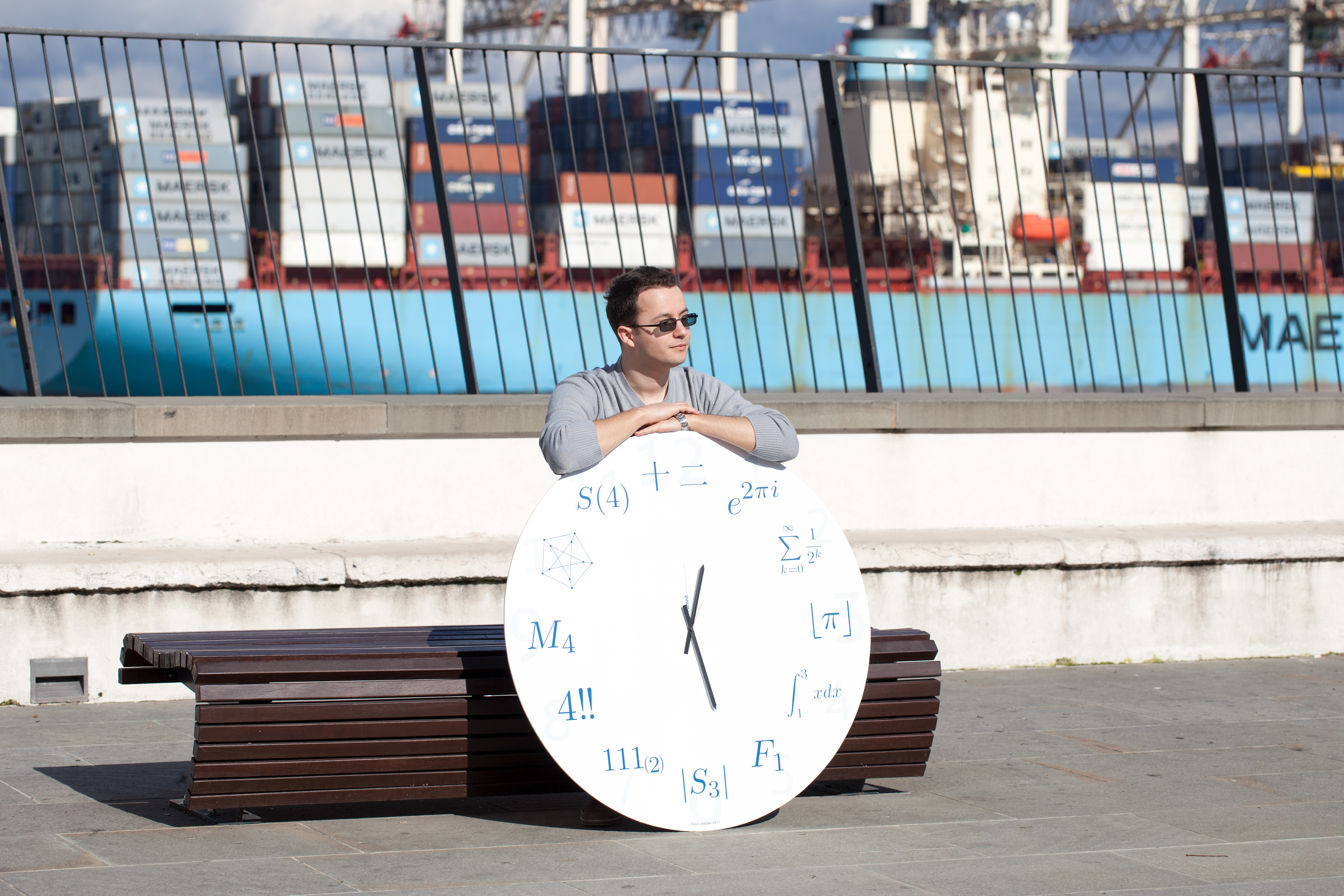SI | EN
natisni

Köhler Ekkehard, Universität Cottbus, Germany.

Title: Linear structure of graphs and the knotting graph

Abstract: Many important graph classes, as interval graphs, comparability graphs
and AT-free graphs, show some kind of linear structure.  In this talk
we try to capture the notion of linearity and show some algorithmic
implications.

In the first part of the talk we discuss the notion of linearity of
graphs and give some motivation for its usefulness for particular
graph classes.  Then we consider the knotting graph, a combinatorial
structure that was defined by Gallai long ago and that has various
nice properties with respect to our notion of linearity. Next we
define intervals of graphs, a concept that generalizes betweenness in
graphs--a crucial notion for capturing linear structure in graphs.  In
the last part we give a practical example of how to use linear
structure of graphs algorithmically. In particular we show how to use
these structural insights for finding maximum independent sets in
AT-free graphs in O(nm') time, where m' denotes the number of
non-edges of the graph G.